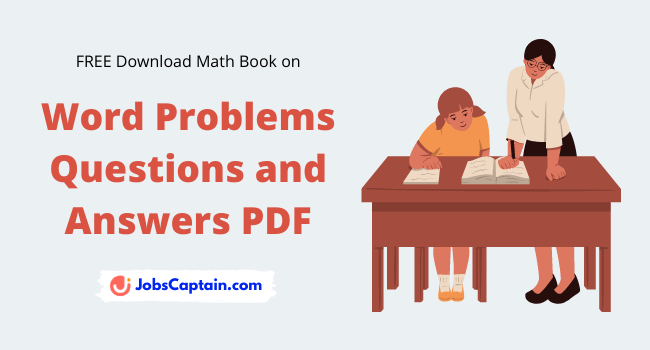Word Problems Questions and Answers PDF

By | December 24, 2021Let’s access the answers to hundreds of Math Word Problems questions with answers PDF that is explained in a way that’s easy for you to understand.

Contents

Fraction Word Problems PDF

In this section we covered the following topics of Fraction:

• Introduction to Fractions
• Reducing Fractions
• Ordering Fractions
• Multiplication and Division of Fractions
• Addition and Subtraction of Fractions